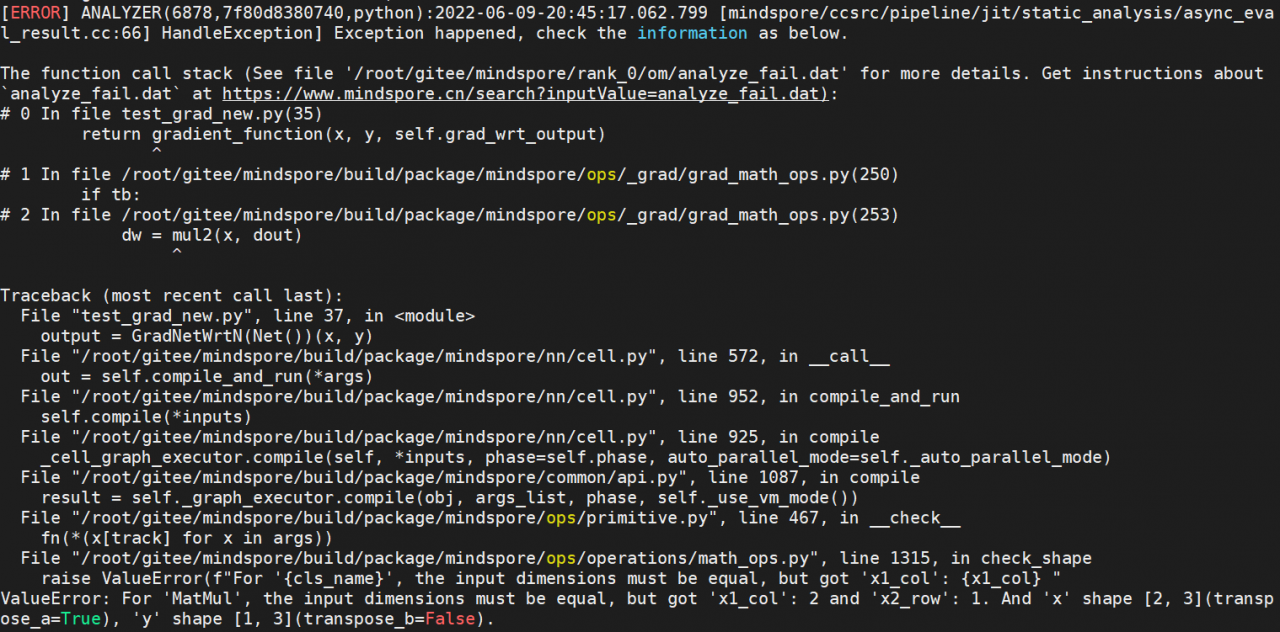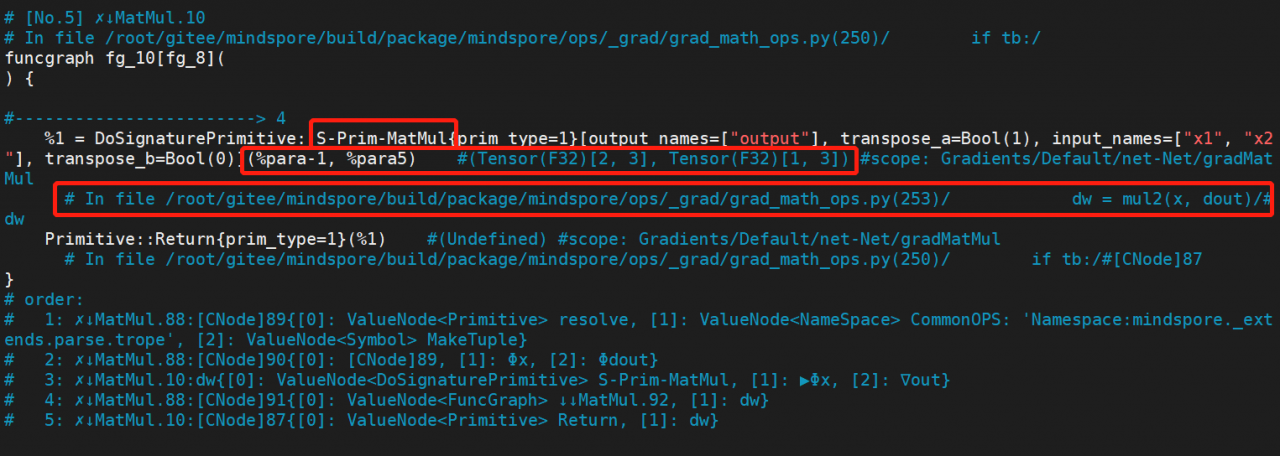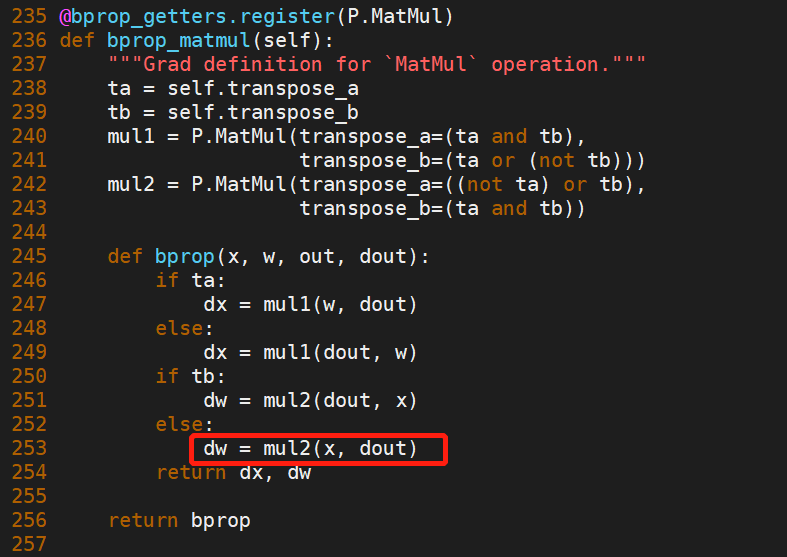# 1 Error description

## 1.1 System Environment

Hardware Environment(Ascend/GPU/CPU): GPU
Software Environment:

• MindSpore version (source or binary): 1.7.0
• Python version (e.g., Python 3.7.5): 3.7.5
• OS platform and distribution (e.g., Linux Ubuntu 16.04): Ubuntu 18.04.4 LTS
• GCC/Compiler version (if compiled from source): 7.5.0

## 1.2 Basic information

### 1.2.1 Source code

``````import numpy as np
import mindspore.nn as nn
import mindspore.ops as ops
from mindspore import Tensor
from mindspore import ParameterTuple, Parameter
from mindspore import dtype as mstype

x = Tensor([[0.8, 0.6, 0.2], [1.8, 1.3, 1.1]], dtype=mstype.float32)
y = Tensor([[0.11, 3.3, 1.1], [1.1, 0.2, 1.4], [1.1, 2.2, 0.3]], dtype=mstype.float32)

class Net(nn.Cell):
def __init__(self):
super(Net, self).__init__()
self.matmul = ops.MatMul()
self.z = Parameter(Tensor(np.array([1.0], np.float32)), name='z')

def construct(self, x, y):
x = x * self.z
out = self.matmul(x, y)
return out

def __init__(self, net):
self.net = net
self.grad_wrt_output = Tensor([[0.1, 0.6, 0.2]], dtype=mstype.float32)

def construct(self, x, y):

print(output)
``````

### 1.2.2 Error reporting# 2 Reason analysis

1. According to the error message, the MatMul operator checks that the input shape is incorrect when infer shape, specifically the number of columns of x1 is not equal to the number of rows of x2.
2. Open the debug file provided by the error report `/root/gitee/mindspore/rank_0/om/analyze_fail.dat`, and the interception part is as follows:Refer to the analysis_fail.dat file analysis guide , it can be seen that the first red box of MatMul reports an error in the infer shape. Then look at the second red box. The shape of the first input of the operator is (2, 3), and the shape of the second input is (1, 3), which is consistent with the error message (note the transpose_a attribute of MatMul here. is True). Finally, let’s look at the third red box. The MatMul is `grad_math_ops.py`called in line 253 of the file. It is the operator generated by the back-propagation rule of the MatMul operator. The back-propagation rule of the MatMul operator is as follows:Let’s see The shape of the two inputs to this MatMul operator, namely `x`and `dout``x`is confirmed to be correct, that is `dout`, the shape is wrong.
3. From the mechanism of reverse automatic differentiation, we know that the first operator of the reverse part is generated from the back propagation rule of the last operator of the forward part. The forward network has only one MatMul operator, and it is the last operator, so the reverse MatMul operator reported by the infer shape error is generated from the back propagation rule of this forward MatMul operator (this use case It is relatively simple. There is only one MatMul operator in the forward network. Combine the input and output of the operator to infer from which forward operator a reverse operator is backpropagated. rule-generated), and is the first operator of the reverse part. Therefore, the second input of this reverse MatMul `dout`can only be passed in from the outside, that is, the self.grad_wrt_output passed in the use case. That is, the shape of self.grad_wrt_output is wrong.

# 3 Solutions

The sens value passed in by GradOperation is the gradient of the forward network output passed by the script from the outside, which can play the role of gradient value scaling. Since it is about the gradient output of the forward network, the shape of the sens value needs to be consistent with the output shape of the forward network (which can be obtained by calling the forward network and printing its output shape). Let’s change the value of self.grad_wrt_output in the above use case, as follows:

``````self.grad_wrt_output = Tensor([[0.1, 0.6, 0.2], [0.8, 1.3, 1.1]], dtype=mstype.float32)
``````

Finally the problem can be solved.